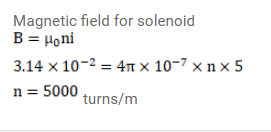# The magnetic field B inside a long solenoid,

Question:

The magnetic field $\mathrm{B}$ inside a long solenoid, carrying a current of $5.00 \mathrm{~A}$, is $3.14 \times 10^{-2} \mathrm{~T}$. Find the number of turns per unit length of the solenoid.

Solution: